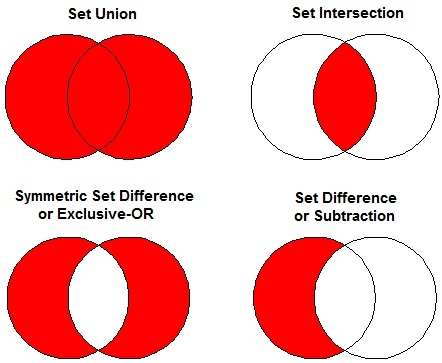# C++ Program to Implement Set_Union in STL

C++Server Side ProgrammingProgramming

The union of two sets is created by the elements that are present in either one of the sets, or in both. Elements from the second set that has the same element in the first set are not copied to the resulting set.

Common set operations are −

• Set Union
• Set Intersection
• Symmetric Set Difference or Exclusive-OR
• Set Difference or Subtraction## Algorithm

Begin
Declare set vector v and iterator st.
Initialize st= set_union (set1, set1 + n, set2, set2 +n, v.begin()))
Print the elements.
End.

## Example Code

Live Demo

#include <iostream>
#include <algorithm>
#include <vector>
using namespace std;
int main () {
int set1[] = {5,6,7,8,9,10};
int set2[] = {1,2,3,4,6,7};
vector<int> v(10);
vector<int>::iterator st;
sort (set1, set1 + 6);
sort (set2, set2 + 6);
st = set_union(set1, set1 + 6, set2, set2 + 6, v.begin());
v.resize(st - v.begin());
cout<<"The union between the sets has "<< (v.size())<< " elements: "<<endl;
for (st = v.begin(); st != v.end(); ++st)
cout<< *st<<" ";
cout <<endl;
return 0;
}

## Output

The union between the sets has 10 elements:
1 2 3 4 5 6 7 8 9 10